# Multi-Layer Perceptron Digit-Classifier using TensorFlow

## Multi-layer Perceptron Digit-Classifier using TensorFlow

In this article, I am going to discuss Multi-layer Perceptron Digit-Classifier using TensorFlow. Please read our previous article where we discussed Building Multi-Layer Perceptron Neural Network Models.

##### Multi-layer Perceptron Digit-Classifier using TensorFlow

Multi-layer Perceptron (MLP) is the abbreviation for multi-layer perception. It is made up of dense, completely connected layers that may change any input dimension into the desired dimension. A neural network with numerous layers is referred to as a multi-layer perception. In order to build a neural network, we combine neurons so that some of their outputs are also their inputs.

Let’s start by writing some Python code to use the TensorFlow library to build a model for the Digit Classifier based on the MNIST dataset.

###### 1. Import Necessary Libraries
```import numpy as np
import matplotlib.pyplot as plt
import seaborn as sns
import tensorflow
import keras
```
###### 2. Import the Dataset

We can read the MNIST dataset using TensorFlow and load it directly into the application as a train and test dataset.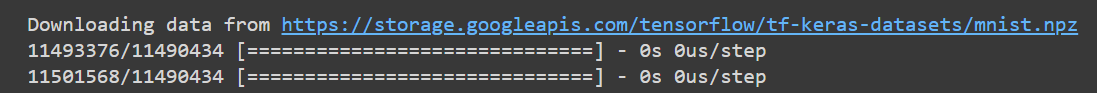###### 3. Data Exploration

Let’s see what this data looks like.

X_train.shape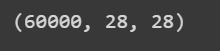y_train.shape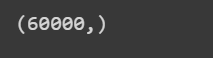plt.imshow(X_train)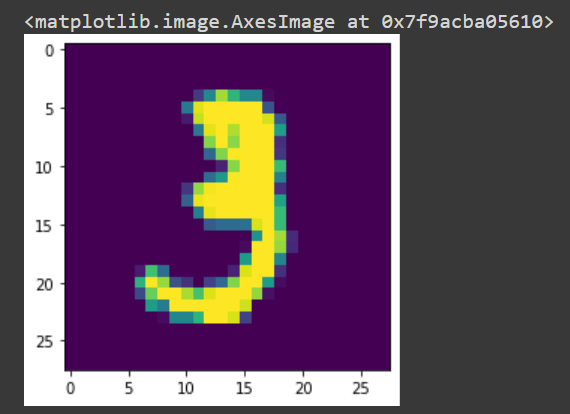###### 4. Data Preprocessing

To make the predictions, we translate the pixel data into floating-point numbers. As the numbers become smaller and the computation becomes simpler and faster, changing the integers into grayscale values will be advantageous. Since pixel values vary from 0 to 256, the range is 255 except for 0. Thus, multiplying all of the numbers by 255 will change the range to be from 0 to 1.

```# Normalization of all values between 0 and 1 (to grayscale)
X_train = X_train/255
X_test = X_test/255
```
###### 5. Model Building
1. The Sequential model is restricted to single-input, single-output stacks of layers and allows us to build models layer by layer as needed in a multi-layer perceptron.
2. Without altering the batch size, flatten flattens the input that is given. For instance, flattening adds an additional channel dimension and produces an output shape of (batch size, 1) if inputs are shaped as (batch size,) without a feature axis.
3. The sigmoid activation function requires activation.
4. The first two dense layers—which are also the hidden layers—are used to create a fully connected model.
5. The output layer, the final densest layer, comprises 10 neurons that select the category to which the image belongs.
```# Model Building
from tensorflow.keras.models import Sequential
from tensorflow.keras.layers import Flatten
from tensorflow.keras.layers import Dense
from tensorflow.keras.layers import Activation
model = Sequential()
# reshape into 1-dimensional data

# dense layer 1

# dense layer 2

# output layer

# Model summary
model.summary()
```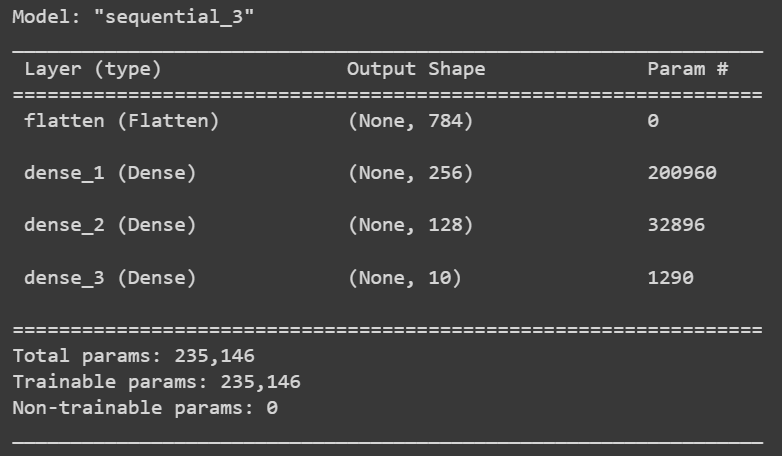```model.compile(loss="sparse_categorical_crossentropy",
model.fit(X_train,y_train,epochs=25,validation_split=0.2)
results = model.evaluate(X_test,  y_test, verbose = 0)
print('test loss, test acc:', results)
```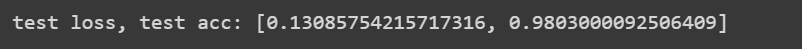###### 6. Model Prediction

Now, let’s try predicting digits from the test data itself to check how accurate this model is performing.

plt.imshow(X_test)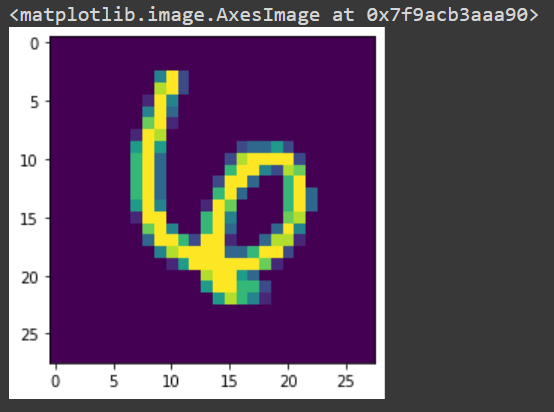model.predict(X_test.reshape(1,28,28)).argmax(axis=1)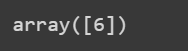In the next article, I am going to give a brief Introduction to Deep Learning and AI. Here, in this article, I try to explain Multi-Layer Perceptron Digit-Classifier using TensorFlow. I hope you enjoy this Multi-Layer Perceptron Digit-Classifier using the TensorFlow article. Please post your feedback, suggestions, and questions about this Multi-Layer Perceptron Digit-Classifier using the TensorFlow article.